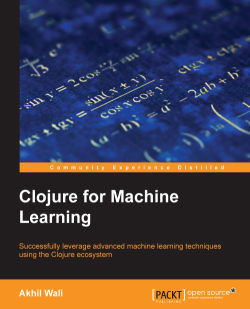### Clojure for Machine Learning1 (1 reviews total)
By Akhil Wali

• Constantly updated with 100+ new titles each month
• Breadth and depth in over 1,000+ technologies

Clojure for Machine Learning is an introduction to machine learning techniques and algorithms. This book demonstrates how you can apply these techniques to real-world problems using the Clojure programming language.

It explores many machine learning techniques and also describes how to use Clojure to build machine learning systems. This book starts off by introducing the simple machine learning problems of regression and classification. It also describes how you can implement these machine learning techniques in Clojure. The book also demonstrates several Clojure libraries, which can be useful in solving machine learning problems.

Clojure for Machine Learning familiarizes you with several pragmatic machine learning techniques. By the end of this book, you will be fully aware of the Clojure libraries that can be used to solve a given machine learning problem.

Publication date:
April 2014
Publisher
Packt
Pages
292
ISBN
9781783284351

## Chapter 1. Working with Matrices

In this chapter, we will explore an elementary yet elegant mathematical data structure—the matrix. Most computer science and mathematics graduates would already be familiar with matrices and their applications. In the context of machine learning, matrices are used to implement several types of machine-learning techniques, such as linear regression and classification. We will study more about these techniques in the later chapters.

Although this chapter may seem mostly theoretical at first, we will soon see that matrices are a very useful abstraction for quickly organizing and indexing data with multiple dimensions. The data used by machine-learning techniques contains a large number of sample values in several dimensions. Thus, matrices can be used to store and manipulate this sample data.

An interesting application that uses matrices is Google Search, which is built on the PageRank algorithm. Although a detailed explanation of this algorithm is beyond the scope of this book, it's worth knowing that Google Search essentially finds the eigen-vector of an extremely massive matrix of data (for more information, refer to The Anatomy of a Large-Scale Hypertextual Web Search Engine). Matrices are used for a variety of applications in computing. Although we do not discuss the eigen-vector matrix operation used by Google Search in this book, we will encounter a variety of matrix operations while implementing machine-learning algorithms. In this chapter, we will describe the useful operations that we can perform on matrices.

## Introducing Leiningen

Over the course of this book, we will use Leiningen (http://leiningen.org/) to manage third-party libraries and dependencies. Leiningen, or `lein`, is the standard Clojure package management and automation tool, and has several powerful features used to manage Clojure projects.

To get instructions on how to install Leiningen, visit the project site at http://leiningen.org/. The first run of the `lein` program could take a while, as it downloads and installs the Leiningen binaries when it's run for the first time. We can create a new Leiningen project using the `new` subcommand of `lein`, as follows:

```\$ lein new default my-project
```

The preceding command creates a new directory, `my-project`, which will contain all source and configuration files for a Clojure project. This folder contains the source files in the `src` subdirectory and a single `project.clj` file. In this command, `default` is the type of project template to be used for the new project. All the examples in this book use the preceding `default` project template.

The `project.clj` file contains all the configuration associated with the project and will have the following structure:

```(defproject my-project "0.1.0-SNAPSHOT"
:description "FIXME: write description"
:url "http://example.com/FIXME"
:url "http://www.eclipse.org/legal/epl-v10.html"}
:dependencies [[org.clojure/clojure "1.5.1"]])```

### Tip

You can download the example code files for all Packt books you have purchased from your account at http://www.packtpub.com. If you purchased this book elsewhere, you can visit http://www.packtpub.com/support and register to have the files e-mailed directly to you.

Third-party Clojure libraries can be included in a project by adding the declarations to the vector with the `:dependencies` key. For example, the core.matrix Clojure library package on Clojars (https://clojars.org/net.mikera/core.matrix) gives us the package declaration `[net.mikera/core.matrix "0.20.0"]`. We simply paste this declaration into the `:dependencies` vector to add the core.matrix library package as a dependency for our Clojure project, as shown in the following code:

```  :dependencies [[org.clojure/clojure "1.5.1"]
[net.mikera/core.matrix "0.20.0"]])```

To download all the dependencies declared in the `project.clj` file, simply run the following `deps` subcommand:

```\$ lein deps
```

Leiningen also provides an REPL (read-evaluate-print-loop), which is simply an interactive interpreter that contains all the dependencies declared in the `project.clj` file. This REPL will also reference all the Clojure namespaces that we have defined in our project. We can start the REPL using the following `repl` subcommand of `lein`. This will start a new REPL session:

```\$ lein repl
```

## Representing matrices

A matrix is simply a rectangular array of data arranged in rows and columns. Most programming languages, such as C# and Java, have direct support for rectangular arrays, while others, such as Clojure, use the heterogeneous array-of-arrays representation for rectangular arrays. Keep in mind that Clojure has no direct support for handling arrays, and an idiomatic Clojure code uses vectors to store and index an array of elements. As we will see later, a matrix is represented as a vector whose elements are the other vectors in Clojure.

Matrices also support several arithmetic operations, such as addition and multiplication, which constitute an important field of mathematics known as Linear Algebra. Almost every popular programming language has at least one linear algebra library. Clojure takes this a step ahead by letting us choose from several such libraries, all of which have a single standardized API interface that works with matrices.

The core.matrix library is a versatile Clojure library used to work with matrices. Core.matrix also contains a specification to handle matrices. An interesting fact about core.matrix is that while it provides a default implementation of this specification, it also supports multiple implementations. The core.matrix library is hosted and developed on GitHub at http://github.com/mikera/core.matrix.

### Note

The core.matrix library can be added to a Leiningen project by adding the following dependency to the `project.clj` file:

`[net.mikera/core.matrix "0.20.0"]`

For the upcoming example, the namespace declaration should look similar to the following declaration:

```(ns my-namespace
(:use clojure.core.matrix))```

Note that the use of `:import` to include library namespaces in Clojure is generally discouraged. Instead, aliased namespaces with the `:require` form are preferred. However, for the examples in the following section, we will use the preceding namespace declaration.

In Clojure, a matrix is simply a vector of vectors. This means that a matrix is represented as a vector whose elements are other vectors. A vector is an array of elements that takes near-constant time to retrieve an element, unlike a list that has linear lookup time. However, in the mathematical context of matrices, vectors are simply matrices with a single row or column.

To create a matrix from a vector of vectors, we use the following `matrix` function and pass a vector of vectors or a quoted list to it. Note that all the elements of the matrix are internally represented as a `double` data type (`java.lang.Double`) for added precision.

```user> (matrix [[0 1 2] [3 4 5]])    ;; using a vector
[[0 1 2] [3 4 5]]
user> (matrix '((0 1 2) (3 4 5)))   ;; using a quoted list
[[0 1 2] [3 4 5]]```

In the preceding example, the matrix has two rows and three columns, or is a 2 x 3 matrix to be more concise. It should be noted that when a matrix is represented by a vector of vectors, all the vectors that represent the individual rows of the matrix should have the same length.

The matrix that is created is printed as a vector, which is not the best way to visually represent it. We can use the `pm` function to print the matrix as follows:

```user> (def A (matrix [[0 1 2] [3 4 5]]))
#'user/A
user> (pm A)
[[0.000 1.000 2.000]
[3.000 4.000 5.000]]```

Here, we define a matrix A, which is mathematically represented as follows. Note that the use of uppercase variable names is for illustration only, as all the Clojure variables are conventionally written in lowercase.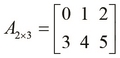The matrix A is composed of elements ai,j where i is the row index and j is the column index of the matrix. We can mathematically represent a matrix A using brackets as follows: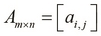We can use the `matrix?` function to check whether a symbol or variable is, in fact, a matrix. The `matrix?` function will return `true` for all the matrices that implement the core.matrix specification. Interestingly, the `matrix?` function will also return `true` for an ordinary vector of vectors.

The default implementation of core.matrix is written in pure Clojure, which does affect performance when handling large matrices. The core.matrix specification has two popular contrib implementations, namely vectorz-clj (http://github.com/mikera/vectorz-clj) that is implemented using pure Java and clatrix (http://github.com/tel/clatrix) that is implemented through native libraries. While there are several other libraries that implement the core.matrix specification, these two libraries are seen as the most mature ones.

### Note

Clojure has three kinds of libraries, namely core, contrib, and third-party libraries. Core and contrib libraries are part of the standard Clojure library. The documentation for both the core and contrib libraries can be found at http://clojure.github.io/. The only difference between the core and contrib libraries is that the contrib libraries are not shipped with the Clojure language and have to be downloaded separately.

Third-party libraries can be developed by anyone and are made available via Clojars (https://clojars.org/). Leiningen supports all of the previous libraries and doesn't make much of a distinction between them.

The contrib libraries are often originally developed as third-party libraries. Interestingly, core.matrix was first developed as a third-party library and was later promoted to a contrib library.

The clatrix library uses the Basic Linear Algebra Subprograms (BLAS) specification to interface the native libraries that it uses. BLAS is also a stable specification of the linear algebra operations on matrices and vectors that are mostly used by native languages. In practice, clatrix performs significantly better than other implementations of core.matrix, and defines several utility functions used to work with matrices as well. You should note that matrices are treated as mutable objects by the clatrix library, as opposed to other implementations of the core.matrix specification that idiomatically treat a matrix as an immutable type.

For most of this chapter, we will use clatrix to represent and manipulate matrices. However, we can effectively reuse functions from core.matrix that perform matrix operations (such as addition and multiplication) on the matrices created through clatrix. The only difference is that instead of using the `matrix` function from the `core.matrix` namespace to create matrices, we should use the one defined in the clatrix library.

### Note

The clatrix library can be added to a Leiningen project by adding the following dependency to the `project.clj` file:

`[clatrix "0.3.0"]`

For the upcoming example, the namespace declaration should look similar to the following declaration:

```(ns my-namespace
(:use clojure.core.matrix)
(:require [clatrix.core :as cl]))```

Keep in mind that we can use both the `clatrix.core` and `clojure.core.matrix` namespaces in the same source file, but a good practice would be to import both these namespaces into aliased namespaces to prevent naming conflicts.

We can create a matrix from the clatrix library using the following `cl/matrix` function. Note that clatrix produces a slightly different, yet more informative representation of the matrix than core.matrix. As mentioned earlier, the `pm` function can be used to print the matrix as a vector of vectors:

```user> (def A (cl/matrix [[0 1 2] [3 4 5]]))
#'user/A
user> A
A 2x3 matrix
-------------
0.00e+00  1.00e+00  2.00e+00
3.00e+00  4.00e+00  5.00e+00
user> (pm A)
[[0.000 1.000 2.000]
[3.000 4.000 5.000]]
nil```

We can also use an overloaded version of the `matrix` function, which takes a matrix implementation name as the first parameter, and is followed by the usual definition of the matrix as a vector, to create a matrix. The implementation name is specified as a keyword. For example, the default persistent vector implementation is specified as `:persistent-vector` and the clatrix implementation is specified as `:clatrix`. We can call the `matrix` function by specifying this keyword argument to create matrices of different implementations, as shown in the following code. In the first call, we call the `matrix` function with the `:persistent-vector` keyword to specify the default persistent vector implementation. Similarly, we call the `matrix` function with the `:clatrix` keyword to create a clatrix implementation.

```user> (matrix :persistent-vector [[1 2] [2 1]])
[[1 2] [2 1]]
user> (matrix :clatrix [[1 2] [2 1]])
A 2x2 matrix
-------------
1.00e+00  2.00e+00
2.00e+00  1.00e+00```

An interesting point is that the vectors of both vectors and numbers are treated as valid parameters for the `matrix` function by clatrix, which is different from how core.matrix handles it. For example, `[0 1]` produces a 2 x 1 matrix, while `[[0 1]]` produces a 1 x 2 matrix. The `matrix` function from core.matrix does not have this functionality and always expects a vector of vectors to be passed to it. However, calling the `cl/matrix` function with either `[0 1]` or `[[0 1]]` will create the following matrices without any error:

```user> (cl/matrix [0 1])
A 2x1 matrix
-------------
0.00e+00
1.00e+00
user> (cl/matrix [[0 1]])
A 1x2 matrix
-------------
0.00e+00  1.00e+00 ```

Analogous to the `matrix?` function, we can use the `cl/clatrix?` function to check whether a symbol or variable is a matrix from the clatrix library. While `matrix?` actually checks for an implementation of the core.matrix specification or protocol, the `cl/clatrix?` function checks for a specific type. If the `cl/clatrix?` function returns `true` for a particular variable, `matrix?` should return `true` as well; however, the converse of this axiom isn't true. If we call `cl/clatrix?` on a matrix created using the `matrix` function and not the `cl/matrix` function, it will return `false`; this is shown in the following code:

```user> (def A (cl/matrix [[0 1]]))
#'user/A
user> (matrix? A)
true
user> (cl/clatrix? A)
true
user> (def B (matrix [[0 1]]))
#'user/B
user> (matrix? B)
true
user> (cl/clatrix? B)
false```

Size is an important attribute of a matrix, and it often needs to be calculated. We can find the number of rows in a matrix using the `row-count` function. It's actually just the length of the vector composing a matrix, and thus, we can also use the standard `count` function to determine the row count of a matrix. Similarly, the `column-count` function returns the number of columns in a matrix. Considering the fact that a matrix comprises equally long vectors, the number of columns should be the length of any inner vector, or rather any row, of a matrix. We can check the return value of the `count`, `row-count`, and `column-count` functions on the following sample matrix in the REPL:

```user> (count (cl/matrix [0 1 2]))
3
user> (row-count (cl/matrix [0 1 2]))
3
user> (column-count (cl/matrix [0 1 2]))
1```

To retrieve an element from a matrix using its row and column indexes, use the following `cl/get` function. Apart from the matrix to perform the operation on, this function accepts two parameters as indexes to the matrix. Note that all elements are indexed relative to 0 in Clojure code, as opposed to the mathematical notation of treating 1 as the position of the first element in a matrix.

```user> (def A (cl/matrix [[0 1 2] [3 4 5]]))
#'user/A
user> (cl/get A 1 1)
4.0
user> (cl/get A 3)
4.0```

As shown in the preceding example, the `cl/get` function also has an alternate form where only a single index value is accepted as a function parameter. In this case, the elements are indexed through a row-first traversal. For example, `(cl/get A 1)` returns `3.0` and `(cl/get A 3)` returns `4.0`. We can use the following `cl/set` function to change an element of a matrix. This function takes parameters similar to `cl/get`—a matrix, a row index, a column index, and lastly, the new element to be set in the specified position in the matrix. The `cl/set` function actually mutates or modifies the matrix it is supplied.

```user> (pm A)
[[0.000 1.0002.000]
[3.000 4.0005.000]]
nil
user> (cl/set A 1 2 0)
#<DoubleMatrix [0.000000, 1.000000, … , 0.000000]>
user> (pm A)
[[0.000 1.000 2.000]
[3.000 4.000 0.000]]
nil```

The clatrix library also provides two handy functions for functional composition: `cl/map` and `cl/map-indexed`. Both these functions accept a function and matrix as arguments and apply the passed function to each element in the matrix, in a manner that is similar to the standard `map` function. Also, both these functions return new matrices and do not mutate the matrix that they are supplied as parameters. Note that the function passed to `cl/map-indexed` should accept three arguments—the row index, the column index, and the element itself:

```user> (cl/map-indexed
(fn [i j m] (* m 2)) A)
A 2x3 matrix
-------------
0.00e+00  2.00e+00  4.00e+00
6.00e+00  8.00e+00  1.00e+01
user> (pm (cl/map-indexed (fn [i j m] i) A))
[[0.000 0.000 0.000]
[1.000 1.000 1.000]]
nil
user> (pm (cl/map-indexed (fn [i j m] j) A))
[[0.000 1.000 2.000]
[0.000 1.000 2.000]]
nil```

## Generating matrices

If the number of rows and columns in a matrix are equal, then we term the matrix as a square matrix. We can easily generate a simple square matrix of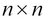size by using the `repeat` function to repeat a single element as follows:

```(defn square-mat
"Creates a square matrix of size n x n
whose elements are all e"
[n e]
(let [repeater #(repeat n %)]
(matrix (-> e repeater repeater))))```

In the preceding example, we define a closure to repeat a value n times, which is shown as the `repeater`. We then use the thread macro (`->`) to pass the element `e` through the closure twice, and finally apply the `matrix` function to the result of the thread macro. We can extend this definition to allow us to specify the matrix implementation to be used for the generated matrix; this is done as follows:

```(defn square-mat
"Creates a square matrix of size n x n whose
elements are all e. Accepts an option argument
for the matrix implementation."
[n e & {:keys [implementation]
:or {implementation :persistent-vector}}]
(let [repeater #(repeat n %)]
(matrix implementation (-> e repeater repeater))))```

The `square-mat` function is defined as one that accepts optional keyword arguments, which specify the matrix implementation of the generated matrix. We specify the default `:persistent-vector` implementation of core.matrix as the default value for the `:implementation` keyword.

Now, we can use this function to create square matrices and optionally specify the matrix implementation when required:

```user> (square-mat 2 1)
[[1 1] [1 1]]
user> (square-mat 2 1 :implementation :clatrix)
A 2x2 matrix
-------------
1.00e+00  1.00e+00
1.00e+00  1.00e+00```

A special type of matrix that's used frequently is the identity matrix. An identity matrix is a square matrix whose diagonal elements are 1 and all the other elements are 0. We formally define an identity matrix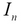as follows: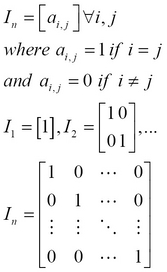We can implement a function to create an identity matrix using the `cl/map-indexed` function that we previously mentioned, as shown in the following code snippet. We first create a square matrix `init` ofsize by using the previously defined `square-mat` function, and then map all the diagonal elements to `1` using `cl/map-indexed`:

```(defn id-mat
"Creates an identity matrix of n x n size"
[n]
(let [init (square-mat :clatrix n 0)
identity-f (fn [i j n]
(if (= i j) 1 n))]
(cl/map-indexed identity-f init)))```

The core.matrix library also has its own version of this function, named `identity-matrix`:

```user> (id-mat 5)
A 5x5 matrix
-------------
1.00e+00  0.00e+00 0.00e+00 0.00e+00 0.00e+00
0.00e+00  1.00e+00 0.00e+00 0.00e+00 0.00e+00
0.00e+00  0.00e+00 1.00e+00 0.00e+00 0.00e+00
0.00e+00  0.00e+00 0.00e+00 1.00e+00 0.00e+00
0.00e+00  0.00e+00 0.00e+00 0.00e+00 1.00e+00
user> (pm (identity-matrix 5))
[[1.000 0.000 0.000 0.000 0.000]
[0.000 1.000 0.000 0.000 0.000]
[0.000 0.000 1.000 0.000 0.000]
[0.000 0.000 0.000 1.000 0.000]
[0.000 0.000 0.000 0.000 1.000]]
nil```

Another common scenario that we will encounter is the need to generate a matrix with random data. Let's implement the following function to generate a random matrix, just like the previously defined `square-mat` function, using the `rand-int` function. Note that the `rand-int` function accepts a single argument `n`, and returns a random integer between `0` and `n`:

```(defn rand-square-mat
"Generates a random matrix of size n x n"
[n]
;; this won't work
(matrix (repeat n (repeat n (rand-int 100))))) ```

But this function produces a matrix whose elements are all single random numbers, which is not very useful. For example, if we call the `rand-square-mat` function with any integer as its parameter, then it returns a matrix with a single distinct random number, as shown in the following code snippet:

```user> (rand-square-mat 4)
[[94 94] [94 94] [94 94] [94 94]]```

Instead, we should map each element of the square matrix generated by the `square-mat` function using the `rand-int` function, to generate a random number for each element. Unfortunately, `cl/map` only works with matrices created by the clatrix library, but we can easily replicate this behavior in Clojure using a lazy sequence, as returned by the `repeatedly` function. Note that the `repeatedly` function accepts the length of a lazily generated sequence and a function to be used as a generator for this sequence as arguments. Thus, we can implement functions to generate random matrices using the clatrix and core.matrix libraries as follows:

```(defn rand-square-clmat
"Generates a random clatrix matrix of size n x n"
[n]
(cl/map rand-int (square-mat :clatrix n 100)))

(defn rand-square-mat
"Generates a random matrix of size n x n"
[n]
(matrix
(repeatedly n #(map rand-int (repeat n 100)))))```

This implementation works as expected, and each element of the new matrix is now an independently generated random number. We can verify this in the REPL by calling the following modified `rand-square-mat` function:

```user> (pm (rand-square-mat 4))
[[97.000 35.000 69.000 69.000]
[50.000 93.000 26.000  4.000]
[27.000 14.000 69.000 30.000]
[68.000 73.000 0.0007 3.000]]
nil
user> (rand-square-clmat 4)
A 4x4 matrix
-------------
5.30e+01  5.00e+00  3.00e+00  6.40e+01
6.20e+01  1.10e+01  4.10e+01  4.20e+01
4.30e+01  1.00e+00  3.80e+01  4.70e+01
3.00e+00  8.10e+01  1.00e+01  2.00e+01```

We can also generate a matrix of random elements using the `cl/rnorm` function from the clatrix library. This function generates a matrix of normally distributed random elements with optionally specified mean and standard deviations. The matrix is normally distributed in the sense that all the elements are distributed evenly around the specified mean value with a spread specified by the standard deviation. Thus, a low standard deviation produces a set of values that are almost equal to the mean.

The `cl/rnorm` function has several overloads. Let's examine a couple of them in the REPL:

```user> (cl/rnorm 10 25 10 10)
A 10x10 matrix
---------------
-1.25e-01  5.02e+01 -5.20e+01  .  5.07e+01  2.92e+01  2.18e+01
-2.13e+01  3.13e+01 -2.05e+01  . -8.84e+00  2.58e+01  8.61e+00
4.32e+01  3.35e+00  2.78e+01  . -8.48e+00  4.18e+01  3.94e+01
...
1.43e+01 -6.74e+00  2.62e+01  . -2.06e+01  8.14e+00 -2.69e+01
user> (cl/rnorm 5)
A 5x1 matrix
-------------
1.18e+00
3.46e-01
-1.32e-01
3.13e-01
-8.26e-02
user> (cl/rnorm 3 4)
A 3x4 matrix
-------------
-4.61e-01 -1.81e+00 -6.68e-01  7.46e-01
1.87e+00 -7.76e-01 -1.33e+00  5.85e-01
1.06e+00 -3.54e-01  3.73e-01 -2.72e-02 ```

In the preceding example, the first call specifies the mean, the standard deviation, and the number of rows and columns. The second call specifies a single argument n and produces a matrix of size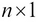. Lastly, the third call specifies the number of rows and columns of the matrix.

The core.matrix library also provides a `compute-matrix` function to generate matrices, and will feel idiomatic to Clojure programmers. This function requires a vector that represents the size of the matrix, and a function that takes a number of arguments that is equal to the number of dimensions of the matrix. In fact, `compute-matrix` is versatile enough to implement the generation of an identity matrix, as well as a matrix of randomly generated elements.

We can implement the following functions to create an identity matrix, as well as a matrix of random elements using the `compute-matrix` function:

```(defn id-computed-mat
"Creates an identity matrix of size n x n
using compute-matrix"
[n]
(compute-matrix [n n] #(if (= %1 %2) 1 0)))

(defn rand-computed-mat
"Creates an n x m matrix of random elements
using compute-matrix"
[n m]
(compute-matrix [n m]
(fn [i j] (rand-int 100))))```

Operations on matrices are not directly supported by the Clojure language but are implemented through the core.matrix specification. Trying to add two matrices in the REPL, as shown in the following code snippet, simply throws an error stating that a vector was found where an integer was expected:

```user> (+ (matrix [[0 1]]) (matrix [[0 1]]))
ClassCastException clojure.lang.PersistentVector cannot be cast to java.lang.Number  clojure.lang.Numbers.add (Numbers.java:126)```

This is because the `+` function operates on numbers rather than matrices. To add matrices, we should use functions from the `core.matrix.operators` namespace. The namespace declaration should look like the following code snippet after we have included `core.matrix.operators`:

```(ns my-namespace
(:use clojure.core.matrix)
(:require [clojure.core.matrix.operators :as M]))```

Note that the functions are actually imported into an aliased namespace, as function names such as `+` and `*` conflict with those in the default Clojure namespace. In practice, we should always try to use aliased namespaces via the `:require` and `:as` filters and avoid the `:use` filter. Alternatively, we could simply not refer to conflicting function names by using the `:refer-clojure` filter in the namespace declaration, which is shown in the following code. However, this should be used sparingly and only as a last resort.

For the code examples in this section, we will use the previous declaration for clarity:

```(ns my-namespace
(:use clojure.core.matrix)
(:require clojure.core.matrix.operators)
(:refer-clojure :exclude [+ - *])) ```

We can use the `M/+` function to perform the matrix addition of two or more matrices. To check the equality of any number of matrices, we use the `M/==` function:

```user> (def A (matrix [[0 1 2] [3 4 5]]))
#'user/A
user> (def B (matrix [[0 0 0] [0 0 0]]))
#'user/B
user> (M/== B A)
false
user> (def C (M/+ A B))
#'user/C
user> C
[[0 1 2] [3 4 5]]
user> (M/== C A)
true```

Two matrices A and B are said to be equal if the following equality holds true: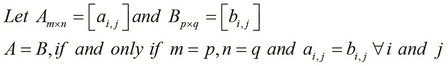Hence, the preceding equation explains that two or more matrices are equal if and only if the following conditions are satisfied:

• Each matrix has the same number of rows and columns

• All elements with the same row and column indices are equal

The following is a simple, yet elegant implementation of matrix equality. It's basically comparing vector equality using the standard `reduce` and `map` functions:

```(defn mat-eq
"Checks if two matrices are equal"
[A B]
(and (= (count A) (count B))
(reduce #(and %1 %2) (map = A B))))```

We first compare the row lengths of the two matrices using the `count` and `=` functions, and then use the `reduce` function to compare the inner vector elements. Essentially, the `reduce` function repeatedly applies a function that accepts two arguments to consecutive elements in a sequence and returns the final result when all the elements in the sequence have been reduced by the applied function.

Alternatively, we could use a similar composition using the `every?` and `true?` Clojure functions. Using the expression `(every? true? (map = A B))`, we can check the equality of two matrices. Keep in mind that the `true?` function returns `true` if it is passed `true` (and `false` otherwise), and the `every?` function returns `true` if a given predicate function returns `true` for all the values in a given sequence.

To add two matrices, they must have an equal number of rows and columns, and the sum is essentially a matrix composed of the sum of elements with the same row and column indices. The sum of two matrices A and B has been formally defined as follows: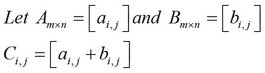It's almost trivial to implement matrix addition using the standard `mapv` function, which is simply a variant of the `map` function that returns a vector. We apply `mapv` to each row of the matrix as well as to the entire matrix. Note that this implementation is intended for a vector of vectors, although it can be easily used with the `matrix` and `as-vec` functions from core.matrix to operate on matrices. We can implement the following function to perform matrix addition using the standard `mapv` function:

```(defn mat-add
[A B]
(mapv #(mapv + %1 %2) A B))```

We can just as easily generalize the `mat-add` function for any number of matrices by using the `reduce` function. As shown in the following code, we can extend the previous definition of `mat-add` to apply it to any number of matrices using the `reduce` function:

```(defn mat-add
([A B]
(mapv #(mapv + %1 %2) A B))
([A B & more]
(let [M (concat [A B] more)]

An interesting unary operation on amatrix A is the trace of a matrix, represented as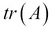. The trace of a matrix is essentially the sum of its diagonal elements: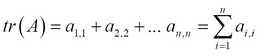It's fairly simple enough to implement the trace function of a matrix using the `cl/map-indexed` and `repeatedly` functions as described earlier. We have skipped it here to serve as an exercise for you.

## Multiplying matrices

Multiplication is another important binary operation on matrices. In the broader sense, the term matrix multiplication refers to several techniques that multiply matrices to produce a new matrix.

Let's define three matrices, A, B, and C, and a single value N, in the REPL. The matrices have the following values: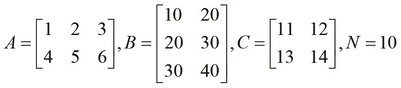We can multiply the matrices by using the `M/*` function from the core.matrix library. Apart from being used to multiply two matrices, this function can also be used to multiply any number of matrices, and scalar values as well. We can try out the following `M/*` function to multiply two given matrices in the REPL:

```user> (pm (M/* A B))
[[140.000 200.000]
[320.000 470.000]]
nil
user> (pm (M/* A C))
RuntimeException Mismatched vector sizes  clojure.core.matrix.impl.persistent-vector/...
user> (def N 10)
#'user/N
user> (pm (M/* A N))
[[10.000 20.000 30.000]
[40.000 50.000 60.000]]
nil```

First, we calculated the product of two matrices. This operation is termed as matrix-matrix multiplication. However, multiplying matrices A and C doesn't work, as the matrices have incompatible sizes. This brings us to the first rule of multiplying matrices: to multiply two matrices A and B, the number of columns in A have to be equal to the number of rows in B. The resultant matrix has the same number of rows as A and columns as B. That's the reason the REPL didn't agree to multiply A and C, but simply threw an exception.

For matrix A of size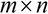, and B of size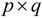, the product of the two matrices only exists if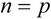, and the product of A and B is a new matrix of size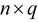.

The product of matrices A and B is calculated by multiplying the elements of rows in A with the corresponding columns in B, and then adding the resulting values to produce a single value for each row in A and each column in B. Hence, the resulting product has the same number of rows as A and columns as B.

We can define the product of two matrices with compatible sizes as follows: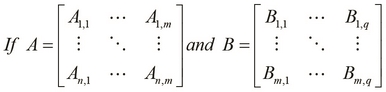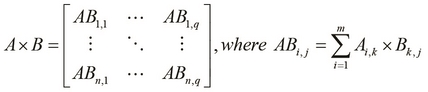The following is an illustration of how the elements from A and B are used to calculate the product of the two matrices: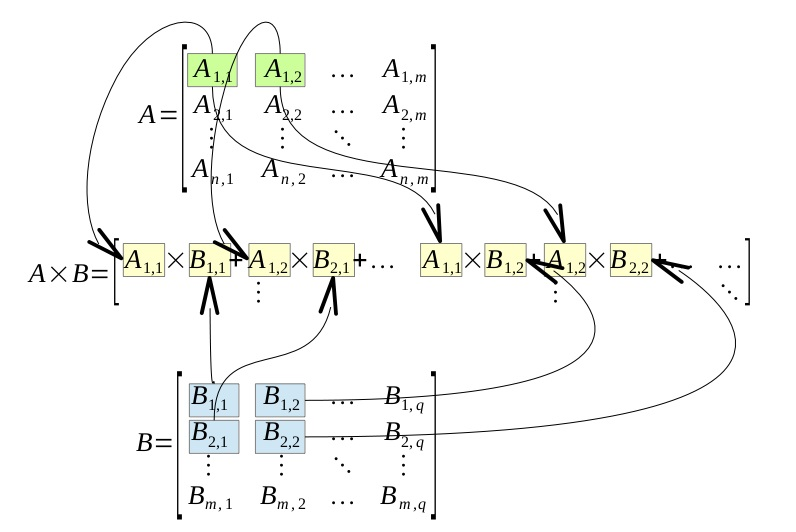This does look slightly complicated, so let's demonstrate the preceding definition with an example, using the matrices A and B as we had previously defined. The following calculation does, in fact, agree to the value produced in the REPL: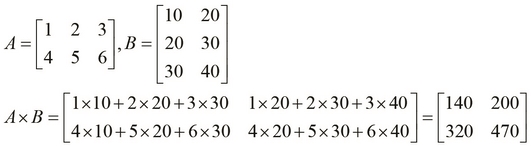Note that multiplying matrices is not a commutative operation. However, the operation does exhibit the associative property of functions. For matrices A, B, and C of product-compatible sizes, the following properties are always true, with one exception that we will uncover later: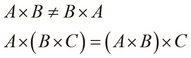An obvious corollary is that a square matrix when multiplied with another square matrix of the same size produces a resultant matrix that has the same size as the two original matrices. Also, the square, cube, and other powers of a square matrix results in matrices of the same size.

Another interesting property of square matrices is that they have an identity element for multiplication, that is, an identity matrix of product-compatible size. But, an identity matrix is itself a square matrix, which brings us to the conclusion that the multiplication of a square matrix with an identity matrix is a commutative operation. Hence, the commutative rule for matrices, which states that matrix multiplication is not commutative, is actually not true when one of the matrices is an identity matrix and the other one is a square matrix. This can be formally summarized by the following equality: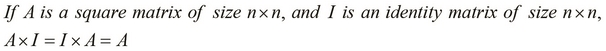A naïve implementation of matrix multiplication would have a time complexity of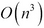, and requires eight multiplication operations for a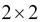matrix. By time complexity, we mean the time taken by a particular algorithm to run till completion. Hence, linear algebra libraries use more efficient algorithms, such as Strassen's algorithm, to implement matrix multiplication, which needs only seven multiplication operations and reduces the complexity to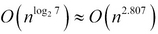.

The clatrix library implementation for matrix multiplication performs significantly better than the default persistent vector implementation, since it interfaces with native libraries. In practice, we can use a benchmarking library such as criterium for Clojure (http://github.com/hugoduncan/criterium) to perform this comparison. Alternatively, we can also compare the performance of these two implementations in brief by defining a simple function to multiply two matrices and then passing large matrices of different implementations to it using our previously defined `rand-square-mat` and `rand-square-clmat` functions. We can define a function to measure the time taken to multiply two matrices.

Also, we can define two functions to multiply the matrices that were created using the `rand-square-mat` and `rand-square-clmat` functions that we previously defined, as follows:

```(defn time-mat-mul
"Measures the time for multiplication of two matrices A and B"
[A B]
(time (M/* A B)))

(defn core-matrix-mul-time []
(let [A (rand-square-mat 100)
B (rand-square-mat 100)]
(time-mat-mul A B)))

(defn clatrix-mul-time []
(let [A (rand-square-clmat 100)
B (rand-square-clmat 100)]
(time-mat-mul A B)))```

We can see that the core.matrix implementation takes a second on average to compute the product of two randomly generated matrices. The clatrix implementation, however, takes less than a millisecond on average, although the first call that's made usually takes 35 to 40 ms to load the native BLAS library. Of course, this value could be slightly different depending on the hardware it's calculated on. Nevertheless, clatrix is preferred when dealing with large matrices unless there's a valid reason, such as hardware incompatibilities or the avoidance of an additional dependency, to avoid its usage.

Next, let's look at scalar multiplication, which involves simply multiplying a single value N or a scalar with a matrix. The resultant matrix has the same size as the original matrix. For a 2 x 2 matrix, we can define scalar multiplication as follows: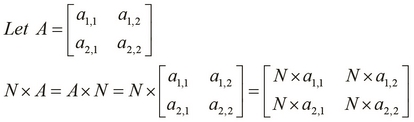For matrices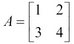and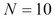, the following is the product: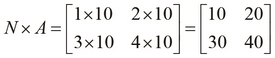Note that we can also use the `scale` function from the core.matrix library to perform scalar multiplication:

```user> (pm (scale A 10))
[[10.000 20.000 30.000]
[40.000 50.000 60.000]]
nil
user> (M/== (scale A 10) (M/* A 10))
true```

Finally, we will briefly take a look at a special form of matrix multiplication, termed as matrix-vector multiplication. A vector is simply a matrix with a single row, which on multiplication with a square matrix of product-compatible size produces a new vector with the same size as the original vector. After multiplying a matrix A of size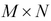and the transpose V' of a vector V, of size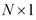, a new vector V" of size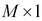is produced. If A is a square matrix, then V" has an identical size as that of the transpose V'.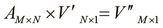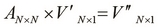## Transposing and inverting matrices

Another frequently used elementary matrix operation is the transpose of a matrix. The transpose of a matrix A is represented as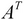or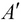. A simple way to define the transpose of a matrix is by reflecting the matrix over its prime diagonal. By prime diagonal, we mean the diagonal comprising elements whose row and column indices are equal. We can also describe the transpose of a matrix by swapping of the rows and columns of a matrix. We can use the following `transpose` function from core.matrix to perform this operation:

```user> (def A (matrix [[1 2 3] [4 5 6]]))
#'user/A
user> (pm (transpose A))
[[1.000 4.000]
[2.000 5.000]
[3.000 6.000]]
nil```

We can define the following three possible ways to obtain the transpose of a matrix:

• The original matrix is reflected over its main diagonal

• The rows of the matrix become the columns of its transpose

• The columns of the matrix become the rows of its transpose

Hence, every element in a matrix has its row and column swapped in its transpose, and vice versa. This can be formally represented using the following equation: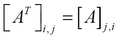This brings us to the notion of an invertible matrix. A square matrix is said to be invertible if there exists another square matrix that is the inverse of a matrix, and which produces an identity matrix when multiplied with the original matrix. A matrix A of size, is said to have an inverse matrix B if the following equality is true: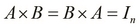Let's test this equality using the `inverse` function from core.matrix. Note that the default persistent implementation of the core.matrix library does not implement the inverse operation, so we use a matrix from the clatrix library instead. In the following example, we create a matrix from the clatrix library using the `cl/matrix` function, determine its inverse using the `inverse` function, and multiply these two matrices using the `M/*` function:

```user> (def A (cl/matrix [[2 0] [0 2]]))
#'user/A
user> (M/* (inverse A) A)
A 2x2 matrix
-------------
1.00e+00  0.00e+00
0.00e+00  1.00e+00```

In the preceding example, we first define a matrix A and then multiply it with its inverse to produce the corresponding identity matrix. An interesting observation when we use double precision numeric types for the elements in a matrix is that not all matrices produce an identity matrix on multiplication with their inverse.

A small amount of error can be observed for some matrices, and this happens due to the limitations of using a 32-bit representation for floating-point numbers; this is shown as follows:

```user> (def A (cl/matrix [[1 2] [3 4]]))
#'user/A
user> (inverse A)
A 2x2 matrix
-------------
-2.00e+00  1.00e+00
1.50e+00 -5.00e-01```

In order to find the inverse of a matrix, we must first define the determinant of that matrix, which is simply another value determined from a given matrix. First off, determinants only exist for square matrices, and thus, inverses only exist for matrices with an equal number of rows and columns. The determinant of a matrix is represented as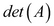or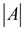. A matrix whose determinant is zero is termed as a singular matrix. For a matrix A, we define its determinant as follows: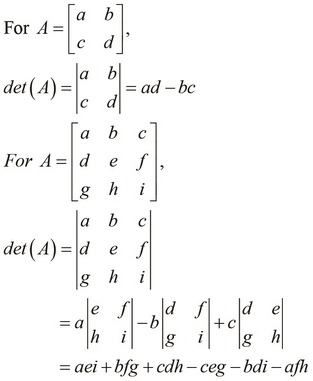We can use the preceding definitions to express the determinant of a matrix of any size. An interesting observation is that the determinant of an identity matrix is always 1. As an example, we will find the determinant of a given matrix as follows: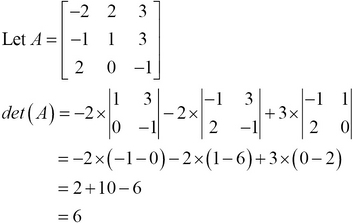For a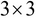matrix, we can use Sarrus' rule as an alternative means to calculate the determinant of a matrix. To find the determinant of a matrix using this scheme, we first write out the first two columns of the matrix to the right of the third column, so that there are five columns in a row. Next, we add the products of the diagonals going from top to bottom, and subtract the products of diagonals from bottom. This process can be illustrated using the following diagram: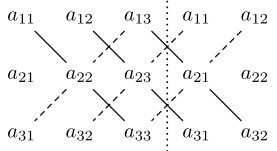By using Sarrus' rule, we formally express the determinant of a matrix A as follows: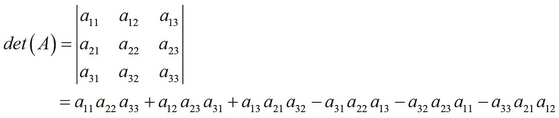We can calculate the determinant of a matrix in the REPL using the following `det `function from core.matrix. Note that this operation is not implemented by the default persistent vector implementation of core.matrix.

```user> (def A (cl/matrix [[-2 2 3] [-1 1 3] [2 0 -1]]))
#'user/A
user> (det A)
6.0```

Now that we've defined the determinant of a matrix, let's use it to define the inverse of a matrix. We've already discussed the notion of an invertible matrix; finding the inverse of a matrix is simply determining a matrix such that it produces an identity matrix when multiplied with the original matrix.

For the inverse of a matrix to exist, its determinant must be nonzero. Next, for each element in the original matrix, we find the determinant of the matrix without the row and column of the selected element. This produces a matrix of an identical size as that of the original matrix (termed as the cofactor matrix of the original matrix). The transpose of the cofactor matrix is called the adjoint of the original matrix. The adjoint produces the inverse on dividing it by the determinant of the original matrix. Now, let's formally define the inverse of amatrix A. We denote the inverse of a matrix A as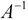, and it can be formally expressed as follows: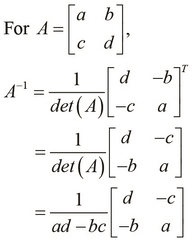As an example, let's find the inverse of a samplematrix. We can actually verify that the inverse produces an identity matrix when multiplied with the original matrix, as shown in the following example: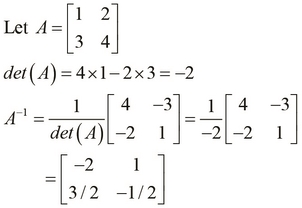Similarly, we define the inverse of amatrix as follows: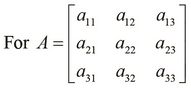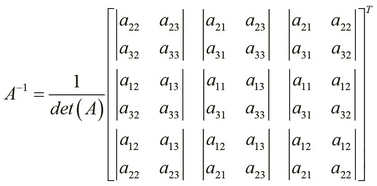Now, let's calculate the inverse of amatrix: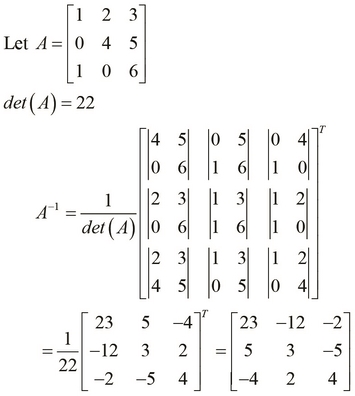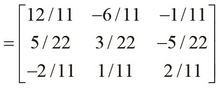We've mentioned that singular and nonsquare matrices don't have inverses, and we can see that the `inverse` function throws an error when supplied with such a matrix. As shown in the following REPL output, the `inverse` function will throw an error if the given matrix is not a square matrix, or if the given matrix is singular:

```user> (def A (cl/matrix [[1 2 3] [4 5 6]]))
#'user/A
user> (inverse A)
ExceptionInfo throw+: {:exception "Cannot invert a non-square matrix."}  clatrix.core/i (core.clj:1033)
user> (def A (cl/matrix [[2 0] [2 0]]))
#'user/A
user> (M/* (inverse A) A)
LapackException LAPACK DGESV: Linear equation cannot be solved because the matrix was singular.  org.jblas.SimpleBlas.gesv (SimpleBlas.java:274)```

## Interpolating using matrices

Let's try out an example to demonstrate how we use matrices. This example uses matrices to interpolate a curve between a given set of points. Suppose we have a given set of points representing some data. The objective is to trace a smooth line between the points in order to produce a curve that estimates the shape of the data. Although the mathematical formulae in this example may seem difficult, we should know that this technique is actually just a form of regularization for a linear regression model, and is termed as Tichonov regularization. For now, we'll focus on how to use matrices in this technique, and we shall revisit regularization in depth in Chapter 2, Understanding Linear Regression.

We will first define an interpolation matrix L that can be used to determine an estimated curve of the given data points. It's essentially the vector [-1, 2, -1] moving diagonally across the columns of the matrix. This kind of a matrix is called a band matrix: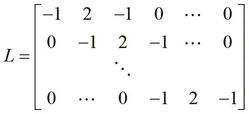We can concisely define the matrix L using the following `compute-matrix` function. Note that for a given size n, we generate a matrix of size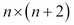:

```(defn lmatrix [n]
(compute-matrix :clatrix [n (+ n 2)]
(fn [i j] ({0 -1, 1 2, 2 -1} (- j i) 0))))```

The anonymous closure in the preceding example uses a map to decide the value of an element at a specified row and column index. For example, the element at row index 2 and column index 3 is 2, since `(- j i)` is 1 and the key 1 in the map has 2 as its value. We can verify that the generated matrix has a similar structure as that of the matrix `lmatrix` through the REPL as follows:

```user> (pm (lmatrix 4))
[[-1.000 2.000 -1.000  0.000  0.000  0.000]
[ 0.000 -1.000  2.000 -1.000  0.000  0.000]
[ 0.000  0.000 -1.000  2.000 -1.000  0.000]
[ 0.000  0.000  0.000 -1.000  2.000 -1.000]]
nil```

Next, we define how to represent the data points that we intend to interpolate over. Each point has an observed value x that is passed to some function to produce another observed value y. For this example, we simply choose a random value for x and another random value for y. We perform this repeatedly to produce the data points.

In order to represent the data points along with an L matrix of compatible size, we define the following simple function named `problem` that returns a map of the problem definition. This comprises the L matrix, the observed values for x, the hidden values of x for which we have to estimate values of y to create a curve, and the observed values for y.

```(defn problem
"Return a map of the problem setup for a
given matrix size, number of observed values
and regularization parameter"
[n n-observed lambda]
(let [i (shuffle (range n))]
{:L (M/* (lmatrix n) lambda)
:observed (take n-observed i)
:hidden (drop n-observed i)
:observed-values (matrix :clatrix
(repeatedly n-observed rand))}))```

The first two parameters of the function are the number of rows `n` in the L matrix, and the number of observed x values `n-observed`. The function takes a third argument `lambda`, which is actually the regularization parameter for our model. This parameter determines how accurate the estimated curve is, and we shall study more about how it's relevant to this model in the later chapters. In the map returned by the preceding function, the observed values for x and y have keys `:observed` and `:observed-values`, and the hidden values for x have the key `:hidden`. Similarly, the key `:L` is mapped to an L matrix of compatible size.

Now that we've defined our problem (or model), we can plot a smooth curve over the given points. By smooth, we mean that each point in the curve is the average of its immediate neighbors, along with some Gaussian noise. Thus, all the points on the curve of this noise have a Gaussian distribution, in which all the values are scattered about some mean value along with a spread specified by some standard deviation.

If we partition matrix L into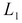and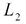over the observed and hidden points respectively, we can define a formula to determine the curve as follows. The following equation may seem a bit daunting, but as mentioned earlier, we shall study the reasoning behind this equation in the following chapters. The curve can be represented by a matrix that can be calculated as follows, using the matrix L: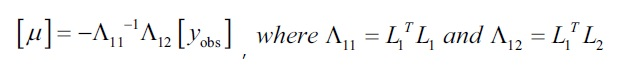We estimate the observed values for the hidden values of x as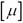, using the originally observed values of y, that is,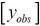and the two matrices that are calculated from the interpolation matrix L. These two matrices are calculated using only the transpose and inverse functions of a matrix. As all the values on the right-hand side of this equation are either matrices or vectors, we use matrix multiplication to find the product of these values.

The previous equation can be implemented using the functions that we've explored earlier. In fact, the code comprises just this equation written as a prefix expression for the map returned by the `problem` function that we defined previously. We now define the following function to solve the problem returned by the `problem` function:

```(defn solve
"Return a map containing the approximated value
y of each hidden point x"
[{:keys [L observed hidden observed-values] :as problem}]
(let [nc  (column-count L)
nr  (row-count L)
L1  (cl/get L (range nr) hidden)
L2  (cl/get L (range nr) observed)
l11 (M/* (transpose L1) L1)
l12 (M/* (transpose L1) L2)]
(assoc problem :hidden-values
(M/* -1 (inverse l11) l12 observed-values))))```

The preceding function calculates the estimated values for y and simply adds them to the original map with the key `:hidden-values` using the `assoc` function.

It's rather difficult to mentally visualize the calculated values of the curve, so we will now use the Incanter library (http://github.com/liebke/incanter) to plot the estimated curve and the original points. This library essentially provides a simple and idiomatic API to create and view various types of plots and charts.

### Note

The Incanter library can be added to a Leiningen project by adding the following dependency to the `project.clj` file:

`[incanter "1.5.4"]`

For the upcoming example, the namespace declaration should look similar to the following:

```(ns my-namespace
[incanter.core   :only [view]])
(:require [clojure.core.matrix.operators :as M]
[clatrix.core :as cl]))```

We now define a simple function that will plot a graph of the given data using functions, such as `xy-plot` and `view`, from the Incanter library:

```(defn plot-points
"Plots sample points of a solution s"
[s]
(let [X (concat (:hidden s) (:observed s))
Y (concat (:hidden-values s) (:observed-values s))]
(view
(xy-plot X Y) (:observed s) (:observed-values s)))))```

As this is our first encounter with the Incanter library, let's discuss some of the functions that are used to implement `plot-points`. We first bind all the values on the x axis to `X`, and all values on the y axis to `Y`. Then, we plot the points as a curve using the `xy-plot` function, which takes two sets of values to plot on the x and y axes as arguments and returns a chart or plot. Next, we add the originally observed points to the plot using the `add-points` function. The `add-points` function requires three arguments: the original plot, a vector of all the values of the x axis component, and a vector of all the values of the y axis component. This function also returns a plot such as the `xy-plot` function, and we can view this plot using the `view` function. Note that we could have equivalently used the thread macro (`->`) to compose the `xy-plot`, `add-points`, and `view` functions.

Now, we can intuitively visualize the estimated curve using the `plot-points` function on some random data as shown in the following function:

```(defn plot-rand-sample []
(plot-points (solve (problem 150 10 30))))```

When we execute the `plot-rand-sample` function, the following plot of the values is displayed: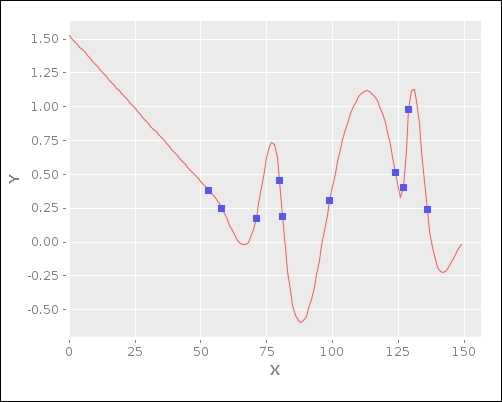## Summary

In this chapter, we introduced matrices through the core.matrix and clatrix libraries. The following are the points that we covered:

• We've discussed how to represent, print, and fetch information from matrices through core.matrix and clatrix. We've also discussed how we can generate matrices with some random data.

• We've talked about some of the rudimentary operations on matrices, such as equality, addition, multiplication, transpose, and inverse.

• We've also introduced the versatile Incanter library that is used to visualize plots and charts of data, through an example on using matrices.

Next, we will study some basic techniques for prediction using linear regression. As we will see, some of these techniques, in fact, are based on simple matrix operations. Linear regression is actually a type of supervised learning, which we will discuss in the next chapter.